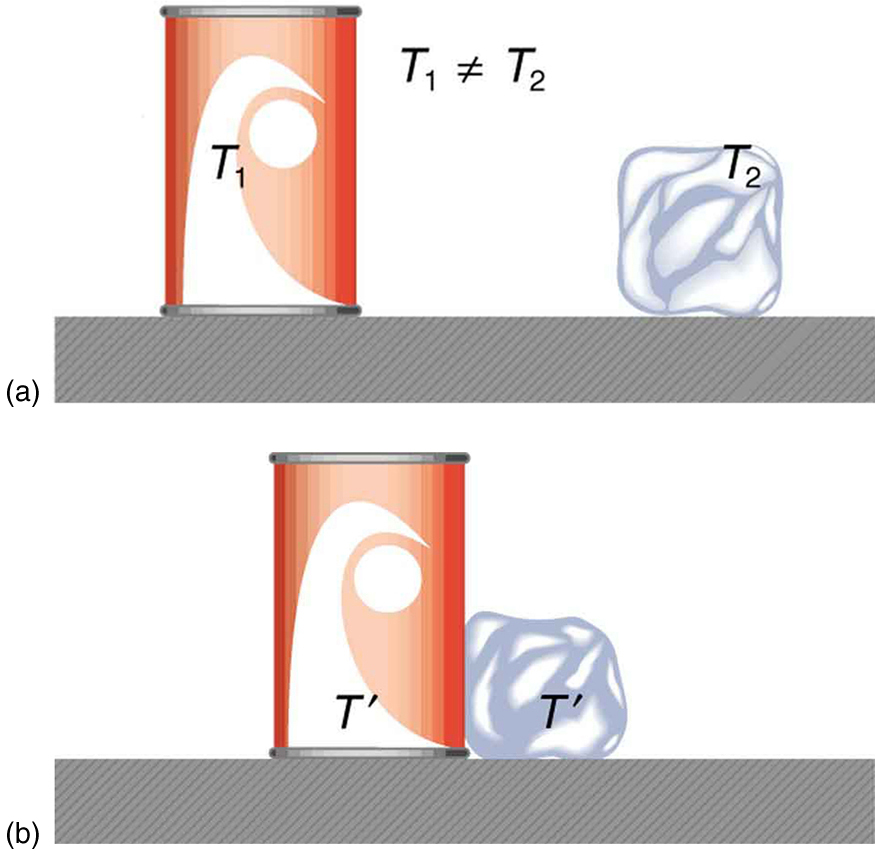Chapter 14 Heat and Heat Transfer Methods

# 100 14.1 Heat

### Summary

• Define heat as transfer of energy.

In Chapter 7 Work, Energy, and Energy Resources, we defined work as force times distance and learned that work done on an object changes its kinetic energy. We also saw in Chapter 13 Temperature, Kinetic Theory, and the Gas Laws that temperature is proportional to the (average) kinetic energy of atoms and molecules. We say that a thermal system has a certain internal energy: its internal energy is higher if the temperature is higher. If two objects at different temperatures are brought in contact with each other, energy is transferred from the hotter to the colder object until equilibrium is reached and the bodies reach thermal equilibrium (i.e., they are at the same temperature). No work is done by either object, because no force acts through a distance. The transfer of energy is caused by the temperature difference, and ceases once the temperatures are equal. These observations lead to the following definition of heat: Heat is the spontaneous transfer of energy due to a temperature difference.

As noted in Chapter 13 Temperature, Kinetic Theory, and the Gas Laws, heat is often confused with temperature. For example, we may say the heat was unbearable, when we actually mean that the temperature was high. Heat is a form of energy, whereas temperature is not. The misconception arises because we are sensitive to the flow of heat, rather than the temperature.

Owing to the fact that heat is a form of energy, it has the SI unit of joule (J). The calorie (cal) is a common unit of energy, defined as the energy needed to change the temperature of 1.00 g of water by$\boldsymbol{1.00^{\circ}\textbf{C}}$ —specifically, between$\boldsymbol{14.5^{\circ}\textbf{C}}$and$\boldsymbol{15.5^{\circ}\textbf{C}},$since there is a slight temperature dependence. Perhaps the most common unit of heat is the kilocalorie (kcal), which is the energy needed to change the temperature of 1.00 kg of water by$\boldsymbol{1.00^{\circ}\textbf{C}}.$Since mass is most often specified in kilograms, kilocalorie is commonly used. Food calories (given the notation Cal, and sometimes called “big calorie”) are actually kilocalories ($\boldsymbol{1\textbf{ kilocalorie }=1000\textbf{ calories}}$), a fact not easily determined from package labeling.Figure 1. In figure (a) the soft drink and the ice have different temperatures, T1 and T2, and are not in thermal equilibrium. In figure (b), when the soft drink and ice are allowed to interact, energy is transferred until they reach the same temperature T′, achieving equilibrium. Heat transfer occurs due to the difference in temperatures. In fact, since the soft drink and ice are both in contact with the surrounding air and bench, the equilibrium temperature will be the same for both.

# Mechanical Equivalent of Heat

It is also possible to change the temperature of a substance by doing work. Work can transfer energy into or out of a system. This realization helped establish the fact that heat is a form of energy. James Prescott Joule (1818–1889) performed many experiments to establish the mechanical equivalent of heatthe work needed to produce the same effects as heat transfer. In terms of the units used for these two terms, the best modern value for this equivalence is

$\boldsymbol{1.000\textbf{ kcal}=4186\textbf{ J}}.$

We consider this equation as the conversion between two different units of energy.

The figure above shows one of Joule’s most famous experimental setups for demonstrating the mechanical equivalent of heat. It demonstrated that work and heat can produce the same effects, and helped establish the principle of conservation of energy. Gravitational potential energy (PE) (work done by the gravitational force) is converted into kinetic energy (KE), and then randomized by viscosity and turbulence into increased average kinetic energy of atoms and molecules in the system, producing a temperature increase. His contributions to the field of thermodynamics were so significant that the SI unit of energy was named after him.

Heat added or removed from a system changes its internal energy and thus its temperature. Such a temperature increase is observed while cooking. However, adding heat does not necessarily increase the temperature. An example is melting of ice; that is, when a substance changes from one phase to another. Work done on the system or by the system can also change the internal energy of the system. Joule demonstrated that the temperature of a system can be increased by stirring. If an ice cube is rubbed against a rough surface, work is done by the frictional force. A system has a well-defined internal energy, but we cannot say that it has a certain “heat content” or “work content”. We use the phrase “heat transfer” to emphasize its nature.

1: Two samples (A and B) of the same substance are kept in a lab. Someone adds 10 kilojoules (kJ) of heat to one sample, while 10 kJ of work is done on the other sample. How can you tell to which sample the heat was added?

# Summary

• Heat and work are the two distinct methods of energy transfer.
• Heat is energy transferred solely due to a temperature difference.
• Any energy unit can be used for heat transfer, and the most common are kilocalorie (kcal) and joule (J).
• Kilocalorie is defined to be the energy needed to change the temperature of 1.00 kg of water between$\boldsymbol{14.5^{\circ}\textbf{C}}$and$\boldsymbol{15.5^{\circ}\textbf{C}}.$
• The mechanical equivalent of this heat transfer is$\boldsymbol{1.00\textbf{ kcal}=4186\textbf{ J}}.$

### Conceptual Questions

1: How is heat transfer related to temperature?

2: Describe a situation in which heat transfer occurs. What are the resulting forms of energy?

3: When heat transfers into a system, is the energy stored as heat? Explain briefly.

## Glossary

heat
the spontaneous transfer of energy due to a temperature difference
kilocalorie
$\boldsymbol{1\textbf{ kilocalorie}=1000\textbf{ calories}}$
mechanical equivalent of heat
the work needed to produce the same effects as heat transfer

### Solutions